Main Content

# pdeplot3D

Plot solution or surface mesh for 3-D problem

## Syntax

``pdeplot3D(model,'ColorMapData',results.NodalSolution)``
``pdeplot3D(model,'ColorMapData',results.Temperature)``
``pdeplot3D(model,'ColorMapData',results.VonMisesStress,'Deformation',results.Displacement)``
``pdeplot3D(model)``
``pdeplot3D(mesh)``
``pdeplot3D(nodes,elements)``
``pdeplot3D(___,Name,Value)``
``h = pdeplot3D(___)``

## Description

example

````pdeplot3D(model,'ColorMapData',results.NodalSolution)` plots the solution at nodal locations as colors on the surface of the 3-D geometry specified in `model`.```

example

````pdeplot3D(model,'ColorMapData',results.Temperature)` plots the temperature at nodal locations for a 3-D thermal analysis model.```

example

````pdeplot3D(model,'ColorMapData',results.VonMisesStress,'Deformation',results.Displacement)` plots the von Mises stress and shows the deformed shape for a 3-D structural analysis model.```

example

````pdeplot3D(model)` plots the surface mesh specified in `model`.```
````pdeplot3D(mesh)` plots the mesh defined as a `Mesh` property of a 3-D `model` object of type `PDEModel`.```
````pdeplot3D(nodes,elements)` plots the mesh defined by `nodes` and `elements`.```

example

````pdeplot3D(___,Name,Value)` plots the surface mesh, the data at nodal locations, or both the mesh and data, depending on the `Name,Value` pair arguments. Use any arguments from the previous syntaxes.```
````h = pdeplot3D(___)` returns a handle to a plot, using any of the previous syntaxes.```

## Examples

collapse all

Plot a PDE solution on the geometry surface. First, create a PDE model and import a 3-D geometry file. Specify boundary conditions and coefficients. Mesh the geometry and solve the problem.

```model = createpde; importGeometry(model,'Block.stl'); applyBoundaryCondition(model,'dirichlet','Face',[1:4],'u',0); specifyCoefficients(model,'m',0,'d',0,'c',1,'a',0,'f',2); generateMesh(model); results = solvepde(model)```
```results = StationaryResults with properties: NodalSolution: [12691x1 double] XGradients: [12691x1 double] YGradients: [12691x1 double] ZGradients: [12691x1 double] Mesh: [1x1 FEMesh] ```

Access the solution at the nodal locations.

`u = results.NodalSolution;`

Plot the solution `u` on the geometry surface.

`pdeplot3D(model,'ColorMapData',u)`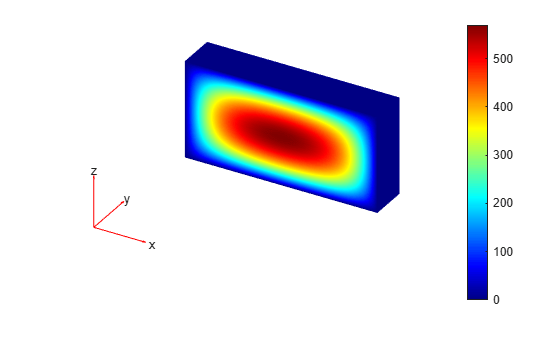Solve a 3-D steady-state thermal problem.

Create a thermal model for this problem.

`thermalmodel = createpde('thermal');`

Import and plot the block geometry.

```importGeometry(thermalmodel,'Block.stl'); pdegplot(thermalmodel,'FaceLabel','on','FaceAlpha',0.5) axis equal```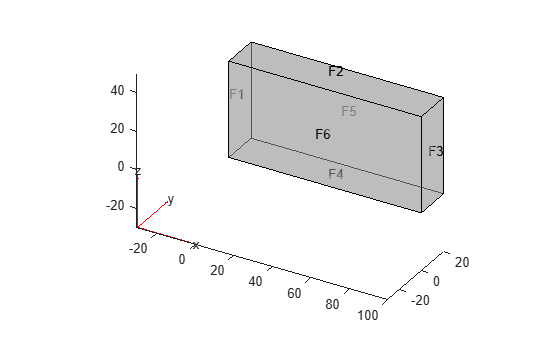Assign material properties.

`thermalProperties(thermalmodel,'ThermalConductivity',80);`

Apply a constant temperature of 100 °C to the left side of the block (face 1) and a constant temperature of 300 °C to the right side of the block (face 3). All other faces are insulated by default.

```thermalBC(thermalmodel,'Face',1,'Temperature',100); thermalBC(thermalmodel,'Face',3,'Temperature',300);```

Mesh the geometry and solve the problem.

```generateMesh(thermalmodel); thermalresults = solve(thermalmodel)```
```thermalresults = SteadyStateThermalResults with properties: Temperature: [12691x1 double] XGradients: [12691x1 double] YGradients: [12691x1 double] ZGradients: [12691x1 double] Mesh: [1x1 FEMesh] ```

The solver finds the temperatures and temperature gradients at the nodal locations. To access these values, use `thermalresults.Temperature`, `thermalresults.XGradients`, and so on. For example, plot temperatures at the nodal locations.

`pdeplot3D(thermalmodel,'ColorMapData',thermalresults.Temperature)`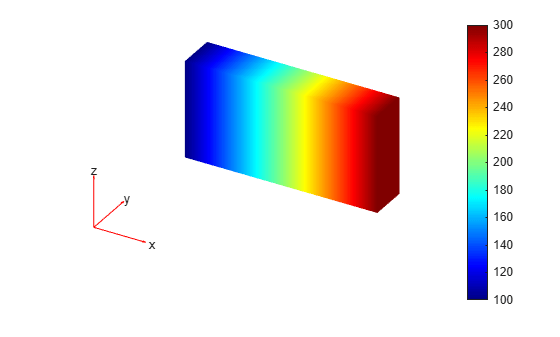For a 3-D steady-state thermal model, evaluate heat flux at the nodal locations and at the points specified by `x`, `y`, and `z` coordinates.

Create a thermal model for steady-state analysis.

`thermalmodel = createpde('thermal');`

Create the following 3-D geometry and include it in the model.

```importGeometry(thermalmodel,'Block.stl'); pdegplot(thermalmodel,'FaceLabels','on','FaceAlpha',0.5) title('Copper block, cm') axis equal```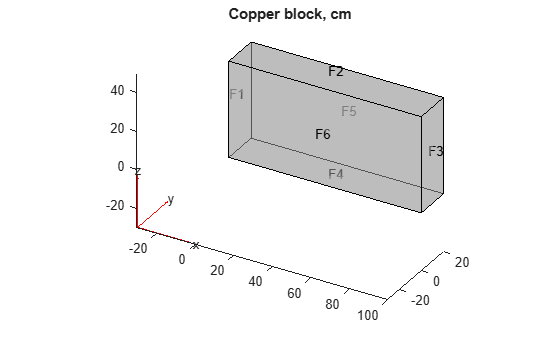Assuming that this is a copper block, the thermal conductivity of the block is approximately $4\phantom{\rule{0.16666666666666666em}{0ex}}W/\left(cmK\right)$.

`thermalProperties(thermalmodel,'ThermalConductivity',4);`

Apply a constant temperature of 373 K to the left side of the block (face 1) and a constant temperature of 573 K to the right side of the block (face 3).

```thermalBC(thermalmodel,'Face',1,'Temperature',373); thermalBC(thermalmodel,'Face',3,'Temperature',573);```

Apply a heat flux boundary condition to the bottom of the block.

`thermalBC(thermalmodel,'Face',4,'HeatFlux',-20);`

Mesh the geometry and solve the problem.

```generateMesh(thermalmodel); thermalresults = solve(thermalmodel)```
```thermalresults = SteadyStateThermalResults with properties: Temperature: [12691x1 double] XGradients: [12691x1 double] YGradients: [12691x1 double] ZGradients: [12691x1 double] Mesh: [1x1 FEMesh] ```

Evaluate heat flux at the nodal locations.

```[qx,qy,qz] = evaluateHeatFlux(thermalresults); figure pdeplot3D(thermalmodel,'FlowData',[qx qy qz])```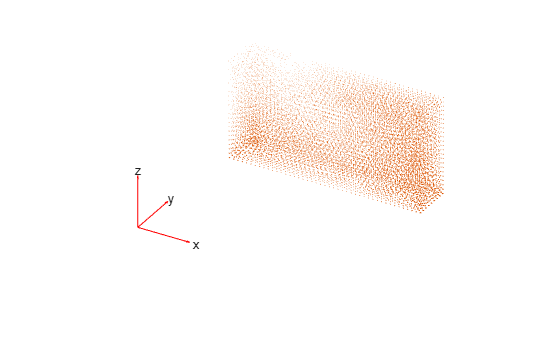Create a grid specified by `x`, `y`, and `z` coordinates, and evaluate heat flux to the grid.

```[X,Y,Z] = meshgrid(1:26:100,1:6:20,1:11:50); [qx,qy,qz] = evaluateHeatFlux(thermalresults,X,Y,Z);```

Reshape the `qx`, `qy`, and `qz` vectors, and plot the resulting heat flux.

```qx = reshape(qx,size(X)); qy = reshape(qy,size(Y)); qz = reshape(qz,size(Z)); figure quiver3(X,Y,Z,qx,qy,qz)```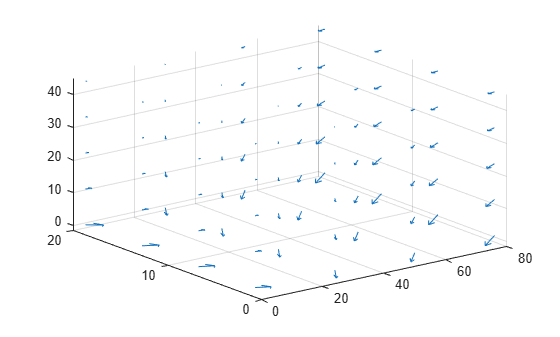Alternatively, you can specify the grid by using a matrix of query points.

```querypoints = [X(:) Y(:) Z(:)]'; [qx,qy,qz] = evaluateHeatFlux(thermalresults,querypoints); qx = reshape(qx,size(X)); qy = reshape(qy,size(Y)); qz = reshape(qz,size(Z)); figure quiver3(X,Y,Z,qx,qy,qz)```Create a structural analysis model for a 3-D problem.

`structuralmodel = createpde('structural','static-solid');`

Import the geometry and plot it.

```importGeometry(structuralmodel,'SquareBeam.STL'); pdegplot(structuralmodel,'FaceLabels','on','FaceAlpha',0.5)```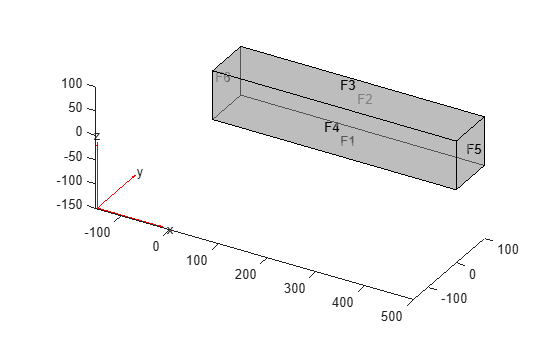Specify the Young's modulus and Poisson's ratio.

```structuralProperties(structuralmodel,'PoissonsRatio',0.3, ... 'YoungsModulus',210E3);```

Specify that face 6 is a fixed boundary.

`structuralBC(structuralmodel,'Face',6,'Constraint','fixed');`

Specify the surface traction for face 5.

`structuralBoundaryLoad(structuralmodel,'Face',5,'SurfaceTraction',[0;0;-2]);`

Generate a mesh and solve the problem.

```generateMesh(structuralmodel); structuralresults = solve(structuralmodel);```

Plot the deformed shape with the von Mises stress using the default scale factor. By default, `pdeplot3D` internally determines the scale factor based on the dimensions of the geometry and the magnitude of deformation.

```figure pdeplot3D(structuralmodel,'ColorMapData',structuralresults.VonMisesStress, ... 'Deformation',structuralresults.Displacement)```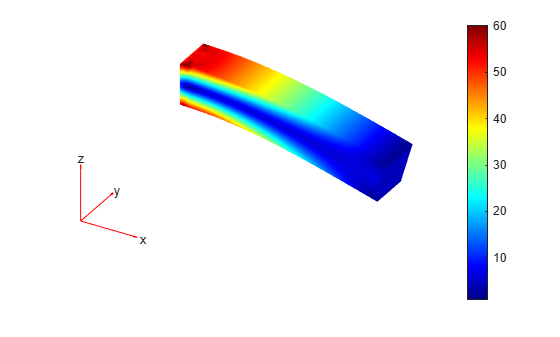Plot the same results with the scale factor 500.

```figure pdeplot3D(structuralmodel,'ColorMapData',structuralresults.VonMisesStress, ... 'Deformation',structuralresults.Displacement, ... 'DeformationScaleFactor',500)```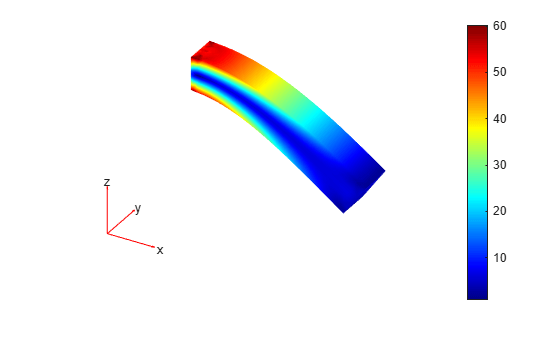Plot the same results without scaling.

```figure pdeplot3D(structuralmodel,'ColorMapData',structuralresults.VonMisesStress)```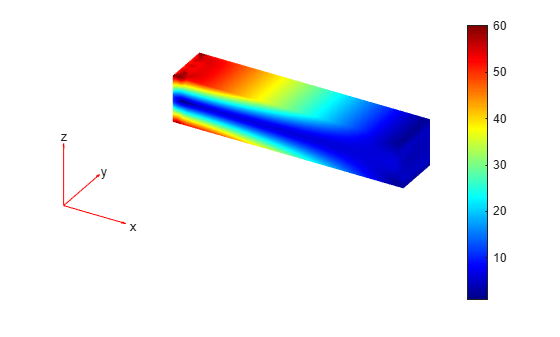Evaluate the von Mises stress in a beam under a harmonic excitation.

Create a transient dynamic model for a 3-D problem.

`structuralmodel = createpde('structural','transient-solid');`

Create the geometry and include it in the model. Plot the geometry.

```gm = multicuboid(0.06,0.005,0.01); structuralmodel.Geometry = gm; pdegplot(structuralmodel,'FaceLabels','on','FaceAlpha',0.5) view(50,20)```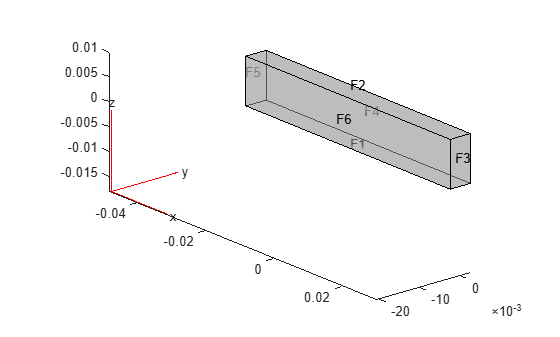Specify the Young's modulus, Poisson's ratio, and mass density of the material.

```structuralProperties(structuralmodel,'YoungsModulus',210E9, ... 'PoissonsRatio',0.3, ... 'MassDensity',7800);```

Fix one end of the beam.

`structuralBC(structuralmodel,'Face',5,'Constraint','fixed');`

Apply a sinusoidal displacement along the y-direction on the end opposite the fixed end of the beam.

`structuralBC(structuralmodel,'Face',3,'YDisplacement',1E-4,'Frequency',50);`

Generate a mesh.

`generateMesh(structuralmodel,'Hmax',0.01);`

Specify the zero initial displacement and velocity.

`structuralIC(structuralmodel,'Displacement',[0;0;0],'Velocity',[0;0;0]);`

Solve the model.

```tlist = 0:0.002:0.2; structuralresults = solve(structuralmodel,tlist);```

Evaluate the von Mises stress in the beam.

`vmStress = evaluateVonMisesStress(structuralresults);`

Plot the von Mises stress for the last time-step.

```figure pdeplot3D(structuralmodel,'ColorMapData',vmStress(:,end)) title('von Mises Stress in the Beam for the Last Time-Step')```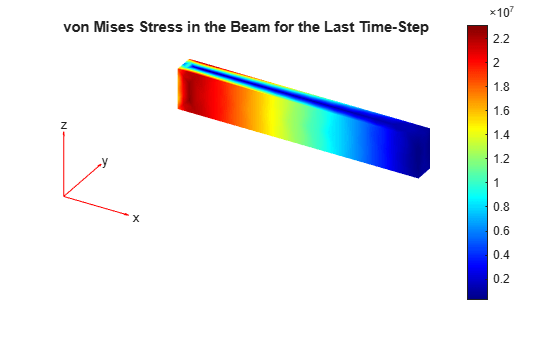Create a PDE model, include the geometry, and generate a mesh.

```model = createpde; importGeometry(model,'Tetrahedron.stl'); mesh = generateMesh(model,'Hmax',20,'GeometricOrder','linear');```

Plot the surface mesh.

`pdeplot3D(model)`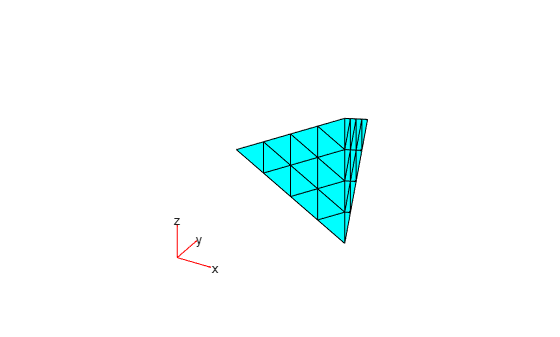Alternatively, you can plot a mesh by using `mesh` as an input argument.

`pdeplot3D(mesh)`Another approach is to use the nodes and elements of the mesh as input arguments for `pdeplot3D`.

`pdeplot3D(mesh.Nodes,mesh.Elements)`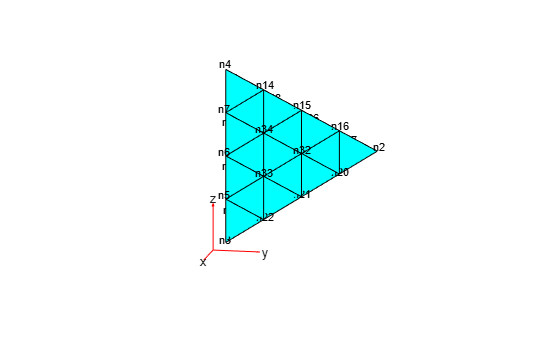Display the node labels on the surface of a simple mesh.

```pdeplot3D(model,'NodeLabels','on') view(101,12)```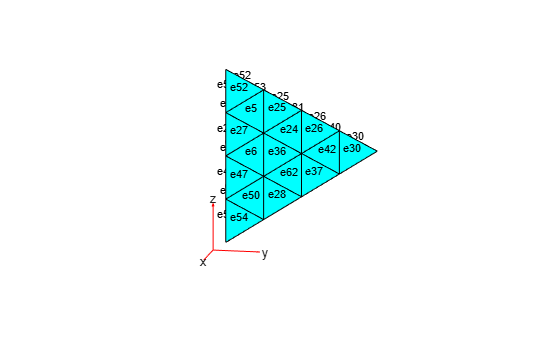Display the element labels.

```pdeplot3D(model,'ElementLabels','on') view(101,12)```## Input Arguments

collapse all

Model object, specified as a `PDEModel` object, `ThermalModel` object, `StructuralModel` object, or `ElectromagneticModel` object.

Example: `model = createpde(3)`

Example: `thermalmodel = createpde('thermal','steadystate')`

Example: `structuralmodel = createpde('structural','static-solid')`

Example: ```emagmodel = createpde('electromagnetic','electrostatic')```

Mesh object, specified as the `Mesh` property of a `PDEModel` object or as the output of `generateMesh`.

Example: `model.Mesh`

Nodal coordinates, specified as a 3-by-NumNodes matrix. NumNodes is the number of nodes.

Element connectivity matrix in terms of the node IDs, specified as a 4-by-NumElements or 10-by-NumElements matrix. Linear meshes contain only corner nodes. For linear meshes, the connectivity matrix has four nodes per 3-D element. Quadratic meshes contain corner nodes and nodes in the middle of each edge of an element. For quadratic meshes, the connectivity matrix has 10 nodes per 3-D element.### Name-Value Pair Arguments

Specify optional comma-separated pairs of `Name,Value` arguments. `Name` is the argument name and `Value` is the corresponding value. `Name` must appear inside quotes. You can specify several name and value pair arguments in any order as `Name1,Value1,...,NameN,ValueN`.

Example: `pdeplot3D(model,'NodeLabels','on')`

Data to plot as a colored surface, specified as the comma-separated pair consisting of `'ColorMapData'` and a column vector with the number of elements that equals the number of points in the mesh. Typically, this data is the solution returned by `solvepde` for a scalar PDE problem and a component of the solution for a multicomponent PDE system.

Example: `'ColorMapData',results.NodalSolution`

Example: `'ColorMapData',results.NodalSolution(:,1)`

Data Types: `double`

Data for the quiver plot, specified as the comma-separated pair consisting of `'FlowData'` and an `M`-by-`3` matrix, where `M` is the number of mesh nodes. `FlowData` contains the x, y, and z values of the field at the mesh points. Set `FlowData` as follows:

```results = solvepde(model); [cgradx,cgrady,cgradz] = evaluateCGradient(results); pdeplot3D(model,'FlowData',[cgradx cgrady cgradz])```

`pdeplot3D` plots the real part of complex data.

Example: ```'FlowData',[cgradx cgrady cgradz]```

Data Types: `double`

Indicator to show the mesh, specified as the comma-separated pair consisting of `'Mesh'` and `'on'` or `'off'`. Specify `'on'` to show the mesh in the plot.

Example: `'Mesh','on'`

Data Types: `char` | `string`

Node labels, specified as the comma-separated pair consisting of `'NodeLabels'` and `'off'` or `'on'`.

Example: `'NodeLabels','on'`

Data Types: `char` | `string`

Element labels, specified as the comma-separated pair consisting of `'ElementLabels'` and `'off'` or `'on'`.

Example: `'ElementLabels','on'`

Data Types: `char` | `string`

Surface transparency for 3-D geometry, specified as the comma-separated pair consisting of `'FaceAlpha'` and a real number from `0` through `1`. The default value `1` indicates no transparency. The value `0` indicates complete transparency.

Example: `'FaceAlpha',0.5`

Data Types: `double`

Deformed shape for structural analysis models, specified as the comma-separated pair consisting of `'Deformation'` and the `FEStruct` object representing displacement values at nodes. The displacement `FEStruct` object is a property of `StaticStructuralResults`, `TransientStructuralResults`, and `FrequencyStructuralResults`.

In an undeformed shape, center nodes in quadratic meshes are always added at half-distance between corners. When you plot a deformed shape, the center nodes might move away from the edge centers.

Example: `'Deformation',results.Displacement`

Scaling factor for plotting the deformed shape, specified as the comma-separated pair consisting of `'DeformationScaleFactor'` and a positive number. Use this argument together with the `Deformation` name-value pair argument. The `pdeplot3D` function chooses the default value based on the geometry itself and on the magnitude of deformation.

Example: `'DeformationScaleFactor',1000`

Data Types: `double`

## Output Arguments

collapse all

Handles to graphics objects, returned as a vector.

## See Also

### Topics

Introduced in R2015a

## SupportGet trial now